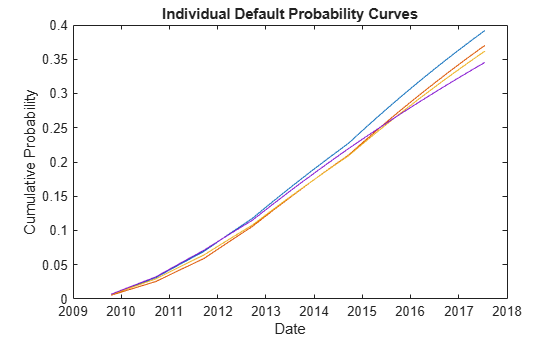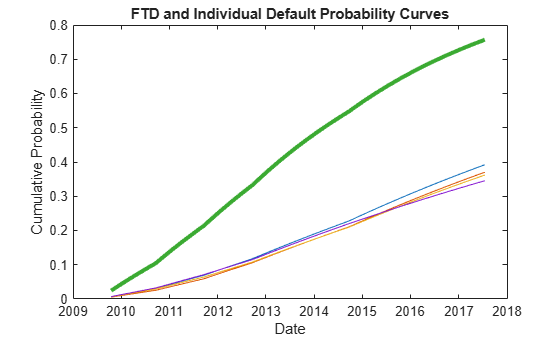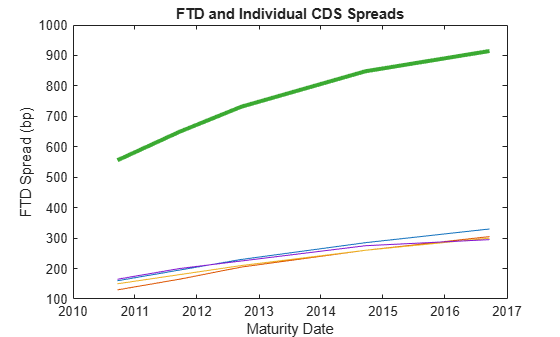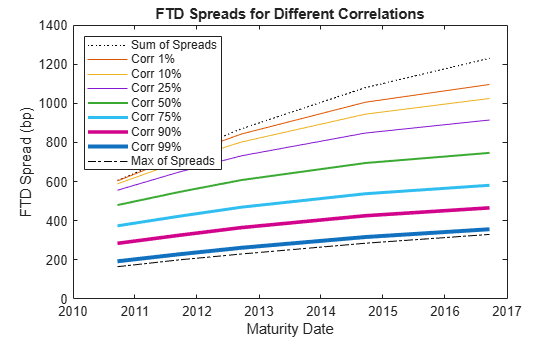Documentation

## First-to-Default Swaps

This example shows how to price first-to-default (FTD) swaps under the homogeneous loss assumption.

A first-to-default swap is an instrument that pays a predetermined amount when (and if) the first of a basket of credit instruments defaults. The credit instruments in the basket are usually bonds. If we assume that the loss amount following a credit event is the same for all credits in the basket, we are under the homogeneous loss assumption. This assumption makes models simpler, because any default in the basket triggers the same payment amount. This example is an implementation of the pricing methodology for these instruments, as described in O'Kane . There are two steps in the methodology: a) Compute the survival probability for the basket numerically; b) Use this survival curve and standard single-name credit-default swap (CDS) functionality to find FTD spreads and to price existing FTD swaps.

### Fit Probability Curves to Market Data

Given CDS market quotes for each issuer in the basket, use `cdsbootstrap` to calibrate individual default probability curves for each issuer.

```% Interest rate curve ZeroDates = datenum({'17-Jan-10','17-Jul-10','17-Jul-11','17-Jul-12',... '17-Jul-13','17-Jul-14'}); ZeroRates = [1.35 1.43 1.9 2.47 2.936 3.311]'/100; ZeroData = [ZeroDates ZeroRates]; % CDS spreads % Each row in MarketSpreads corresponds to a different issuer; each % column to a different maturity date (corresponding to MarketDates) MarketDates = datenum({'20-Sep-10','20-Sep-11','20-Sep-12','20-Sep-14',... '20-Sep-16'}); MarketSpreads = [ 160 195 230 285 330; 130 165 205 260 305; 150 180 210 260 300; 165 200 225 275 295]; % Number of issuers equals number of rows in MarketSpreads nIssuers = size(MarketSpreads,1); % Settlement date Settle = datenum('17-Jul-2009'); ```

In practice, the time axis is discretized and the FTD survival curve is only evaluated at grid points. We use one point every three months. To request that `cdsbootstrap` returns default probability values over the specific grid points we want, use the optional argument 'ProbDates'. We add the original standard CDS market dates to the grid, otherwise the default probability information on those dates would be interpolated using the two closest dates on the grid, and the prices on market dates would be inconsistent with the original market data.

```ProbDates = union(MarketDates,daysadd(Settle,360*(0.25:0.25:8),1)); nProbDates = length(ProbDates); DefProb = zeros(nIssuers,nProbDates); for ii = 1:nIssuers MarketData = [MarketDates MarketSpreads(ii,:)']; ProbData = cdsbootstrap(ZeroData,MarketData,Settle,... 'ProbDates',ProbDates); DefProb(ii,:) = ProbData(:,2)'; end ```

These are the calibrated default probability curves for each credit in the basket.

```figure plot(ProbDates',DefProb) datetick title('Individual Default Probability Curves') ylabel('Cumulative Probability') xlabel('Date') ```### Determine Latent Variable Thresholds

Latent variables are used in different credit risk contexts, with different interpretations. In some contexts, a latent variable is a proxy for a change in the value of assets, and the domain of this variable is binned, with each bin corresponding to a credit rating. The bins limits, or thresholds, are determined from credit migration matrices. In our context, the latent variable is associated to a time to default, and the thresholds determine bins in a discretized time grid where defaults may occur.

Formally, if the time to default of a particular issuer is denoted by, and we know its default probability function, a latent variableand corresponding thresholdssatisfy

``

or

``

These relationships make latent variable approaches convenient for both simulations and analytical derivations. Bothandare functions of time.

The choice of a distribution for the variabledetermines the thresholds. In the standard latent variable model, the variableis chosen to follow a standard normal distribution, from which

``

whereis the cumulative standard normal distribution.

Use the previous formula to determine the default-time thresholds, or simply default thresholds, corresponding to the default probabilities previously obtained for the credits in the basket.

```DefThresh = norminv(DefProb); ```

### Derive Survival Curve for the Basket

Following O'Kane , we use a one-factor latent variable model to derive expressions for the survival probability function of the basket.

Given parametersfor each issuer, and given independent standard normal variablesand, the one-factor latent variable model assumes that the latent variableassociated to issuersatisfies

``

This induces a correlation between issuersandof. All latent variablesshare the common factoras a source of uncertainty, but each latent variable also has an idiosyncratic source of uncertainty. The larger the coefficient, the more the latent variable resembles the common factor.

Using the latent variable model, we derive an analytic formula for the survival probability of the basket.

The probability that issuersurvives past time, in other words, that its default timeis greater thanis

``

whereis the default threshold computed above for issuer, for the-th date in the discretization grid.

Conditional on the value of the one-factor, the probability that all issuers survive past timeis

``

where the product is justified because all the's are independent. Therefore, conditional on, the's are independent.

The unconditional probability of no defaults by timeis the integral over all values ofof the previous conditional probability

``

withthe standard normal density.

By evaluating this one-dimensional integral for each pointin the grid, we get a discretization of the survival curve for the whole basket, which is the FTD survival curve.

The latent variable model can also be used to simulate default times, which is the back engine of many pricing methodologies for credit instruments. Loeffler and Posch , for example, estimate the survival probability of a basket via simulation. In each simulated scenario a time to default is determined for each issuer. With some bookkeeping, the probability of having the first default on each bucket of the grid can be estimated from the simulation. The simulation approach is also discussed in O'Kane . Simulation is very flexible and applicable to many credit instruments. However, analytic approaches are preferred, when available, because they are much faster and more accurate than simulation.

To compute the FTD survival probabilities in our example, we set all betas to the square root of a target correlation. Then we loop over all dates in the time grid to compute the one dimensional integral that gives the survival probability of the basket.

Regarding implementation, the conditional survival probability as a function of a scalar `Z` would be

```condProb=@(Z)prod(normcdf((-DefThresh(:,jj)+beta*Z)./sqrt(1-beta.^2))); ```

However, the integration function we use requires that the function handle of the integrand accepts vectors. Although a loop around the scalar version of the conditional probability would work, it is far more efficient to vectorize the conditional probability using `bsxfun`.

```beta = sqrt(0.25)*ones(nIssuers,1); FTDSurvProb = zeros(size(ProbDates)); for jj = 1:nProbDates % vectorized conditional probability as a function of Z vecCondProb = @(Z)prod(normcdf(bsxfun(@rdivide,... -repmat(DefThresh(:,jj),1,length(Z))+bsxfun(@times,beta,Z),... sqrt(1-beta.^2)))); % truncate domain of normal distribution to [-5,5] interval FTDSurvProb(jj) = integral(@(Z)vecCondProb(Z).*normpdf(Z),-5,5); end FTDDefProb = 1-FTDSurvProb; ```

Compare the FTD probability to the default probabilities of the individual issuers.

```figure plot(ProbDates',DefProb) datetick hold on plot(ProbDates,FTDDefProb,'LineWidth',3) datetick hold off title('FTD and Individual Default Probability Curves') ylabel('Cumulative Probability') xlabel('Date') ```### Find FTD Spreads and Price Existing FTD Swaps

Under the assumption that all instruments in the basket have the same recovery rate, or homogeneous loss assumption (see O'Kane in References), we get the spread for the FTD swap using the `cdsspread` function, but passing the FTD probability data just computed.

```Maturity = MarketDates; ProbDataFTD = [ProbDates, FTDDefProb]; FTDSpread = cdsspread(ZeroData,ProbDataFTD,Settle,Maturity); ```

Compare the FTD spreads with the individual spreads.

```figure plot(MarketDates,MarketSpreads') datetick hold on plot(MarketDates,FTDSpread,'LineWidth',3) hold off title('FTD and Individual CDS Spreads') ylabel('FTD Spread (bp)') xlabel('Maturity Date') ```An existing FTD swap can be priced with `cdsprice`, using the same FTD probability.

```Maturity0 = MarketDates(1); % Assume maturity on nearest market date Spread0 = 540; % Spread of existing FTD contract % Assume default values of recovery and notional FTDPrice = cdsprice(ZeroData,ProbDataFTD,Settle,Maturity0,Spread0); fprintf('Price of existing FTD contract: %g\n',FTDPrice) ```
```Price of existing FTD contract: 17644.7 ```

### Analyze Sensitivity to Correlation

To illustrate the sensitivity of the FTD spreads to model parameters, we calculate the market spreads for a range of correlation values.

```corr = [0 0.01 0.10 0.25 0.5 0.75 0.90 0.99 1]; FTDSpreadByCorr = zeros(length(Maturity),length(corr)); FTDSpreadByCorr(:,1) = sum(MarketSpreads)'; FTDSpreadByCorr(:,end) = max(MarketSpreads)'; for ii = 2:length(corr)-1 beta = sqrt(corr(ii))*ones(nIssuers,1); FTDSurvProb = zeros(length(ProbDates)); for jj = 1:nProbDates % vectorized conditional probability as a function of Z condProb = @(Z)prod(normcdf(bsxfun(@rdivide,... -repmat(DefThresh(:,jj),1,length(Z))+bsxfun(@times,beta,Z),... sqrt(1-beta.^2)))); % truncate domain of normal distribution to [-5,5] interval FTDSurvProb(jj) = integral(@(Z)condProb(Z).*normpdf(Z),-5,5); end FTDDefProb = 1-FTDSurvProb; ProbDataFTD = [ProbDates, FTDDefProb]; FTDSpreadByCorr(:,ii) = cdsspread(ZeroData,ProbDataFTD,Settle,Maturity); end ```

The FTD spreads lie in a band between the sum and the maximum of individual spreads. As the correlation increases to one, the FTD spreads decrease towards the maximum of the individual spreads in the basket (all credits default together). As the correlation decreases to zero, the FTD spreads approach the sum of the individual spreads (independent credits).

```figure legends = cell(1,length(corr)); plot(MarketDates,FTDSpreadByCorr(:,1),'k:') legends{1} = 'Sum of Spreads'; datetick hold on for ii = 2:length(corr)-1 plot(MarketDates,FTDSpreadByCorr(:,ii),'LineWidth',3*corr(ii)) legends{ii} = ['Corr ' num2str(corr(ii)*100) '%']; end plot(MarketDates,FTDSpreadByCorr(:,end),'k-.') legends{end} = 'Max of Spreads'; hold off title('FTD Spreads for Different Correlations') ylabel('FTD Spread (bp)') xlabel('Maturity Date') legend(legends,'Location','NW') ```For short maturities and small correlations, the basket is effectively independent (the FTD spread is very close to the sum of individual spreads). The correlation effect becomes more significant for longer maturities.

Here is an alternative visualization of the dependency of FTD spreads on correlation.

```figure surf(corr,MarketDates,FTDSpreadByCorr) datetick('y') ax = gca; ax.YDir = 'reverse'; view(-40,10) title('FTD Spreads for Different Correlations and Maturities') xlabel('Correlation') ylabel('Maturity Date') zlabel('FTD Spread (bp)') ```### References

 Loeffler, Gunter and Peter Posch, Credit risk modeling using Excel and VBA, Wiley Finance, 2007.

 O'Kane, Dominic, Modelling single-name and multi-name Credit Derivatives, Wiley Finance, 2008.